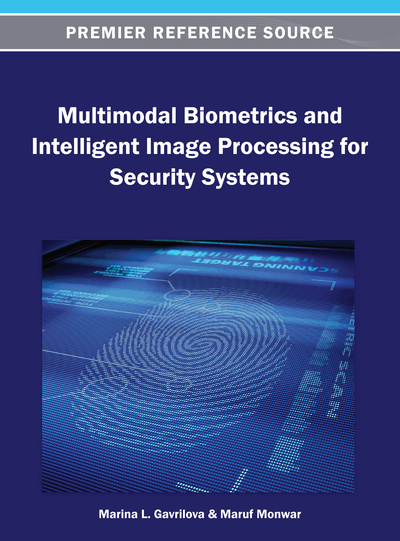# Markov Chain for Multimodal Biometric Rank Fusion

DOI: 10.4018/978-1-4666-3646-0.ch006
Available
\$37.50
No Current Special Offers

## Abstract

Markov chain is a mathematical model used to represent a stochastic process. In this chapter, Markov chain-based rank level fusion method for multimodal biometric authentication system is discussed. Due to some inherent problems associated with existing biometric rank fusion methods, Markov chain-based biometric rank fusion has recently emerged in biometric context. The notion of Markov chain and its construction mechanisms are presented along with discussion on some early research conducted on Markov chain in other rank aggregation frameworks. This chapter also presents a detailed description of recent experimentations conducted to evaluate the performance of Markov chain-based biometric rank fusion method in a face, ear, and iris-based application framework.
Chapter Preview
Top

## 2. Markov Chain

A Markov chain is named for the Russian mathematician Andrei Andreyevich Markov. It is a mathematical model that can be thought of a being in exactly one of a number of states at any time (Markov, 1906). A Markov chain has a set of states, S = {s1; s2;::: ; sr}. The process starts in one of these states and moves successively from one state to another (Kemeny, Snell, & Thompson, 1974). Each move is referred to as a step. If the chain is currently in state si, then it can move to state sj with a probability pij. This probability is preset at the beginning of the process and does not depend on how the state was reached. The probability pij is referred to as transition probabilities. The process can remain in the same state with probability pii. The starting state is given by an initial probability distribution (Kemeny, Snell, & Thompson, 1974).

The following example illustrates how Markov chain operates. Assume that there is a sports team which performance highly depends on its previous history of winning or losing. If the team wins, then there is 50% chance it will win the next game, and 25% chance it will tie or win the next game. If the team ties, there is 75% it will tie again, and 25% it will lose. Finally, if the team loses, there is 50% chance it will lose next game and 50% chance it will win.

Now we can build a Markov chain. .States in this example are W (Win), T(Tie) and L(Lose) . Transitional probabilities can be represented in a matrix:

(6.1)

The entries in the first row of the matrix P in this example represent the probabilities for the win, tie or lose of the team during the next game. The entries in the second and the third row represent probabilities of win, lose or tie following the tie (second row) or the loss (third row) Such an array is commonly called the matrix of transition probabilities, or the transition matrix.

The matrix allows to determine, given the state i, the probability of win, loss or tie in one, two, or any number of consequent games in the future.

## Complete Chapter List

Search this Book:
Reset